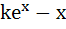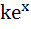Question 192

If a continuous function f defined on the real line ℝ, assumes positive and negative values in ℝ then the equation f(x) = 0 has a root in ℝ. For example, it is known that if a continuous function f on ℝ is positive at some points and its minimum value is negative then the equation f(x) = 0 has a root in ℝ. Consider the function f(x) =for all real x where k is a real constant.

The line y = x meets y =for k ≤ 0 at

a) No point

b) One point

c) Two points

d) More than two points

IIT 2007